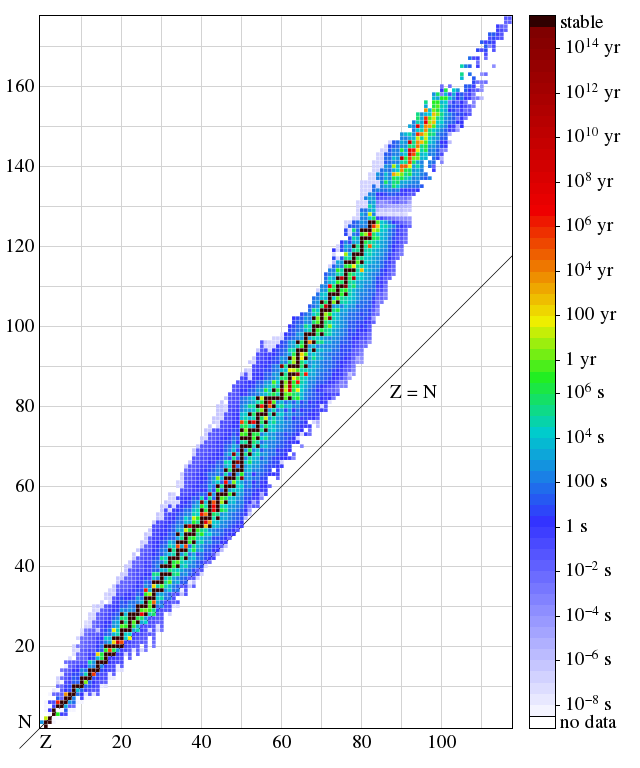# Increasing stability of atomic nuclei

I have issues with your claim that atomic nuclei get more stable if you keep piling up neutrons (in the nuclear energy part of everyday physics). Given the theoretical model of atomic nuclei that you present there, it might make it seem logical, but it's still false. As is quite evident from tables like this one: table of nuclides, nuclei tend to be most stable with approximately the same number of neutrons and protons.

Using simplified models of nuclei is fine, but you shouldn't make false claims based on it. Either just omit the question of which amount of neutrons maximizes stability, or admit that your model is simplified and doesn't always give correct answers. Brilliant.org is said to teach people to think like scientists. Stubbornly believing a theory even when it disagrees with hard facts of reality, is hardly to think like a scientist.Note by Erik Edelmann
3 years, 1 month ago

This discussion board is a place to discuss our Daily Challenges and the math and science related to those challenges. Explanations are more than just a solution — they should explain the steps and thinking strategies that you used to obtain the solution. Comments should further the discussion of math and science.

When posting on Brilliant:

• Use the emojis to react to an explanation, whether you're congratulating a job well done , or just really confused .
• Ask specific questions about the challenge or the steps in somebody's explanation. Well-posed questions can add a lot to the discussion, but posting "I don't understand!" doesn't help anyone.
• Try to contribute something new to the discussion, whether it is an extension, generalization or other idea related to the challenge.

MarkdownAppears as
*italics* or _italics_ italics
**bold** or __bold__ bold
- bulleted- list
• bulleted
• list
1. numbered2. list
1. numbered
2. list
Note: you must add a full line of space before and after lists for them to show up correctly
paragraph 1paragraph 2

paragraph 1

paragraph 2

[example link](https://brilliant.org)example link
> This is a quote
This is a quote
    # I indented these lines
# 4 spaces, and now they show
# up as a code block.

print "hello world"
# I indented these lines
# 4 spaces, and now they show
# up as a code block.

print "hello world"
MathAppears as
Remember to wrap math in $$ ... $$ or $ ... $ to ensure proper formatting.
2 \times 3 $2 \times 3$
2^{34} $2^{34}$
a_{i-1} $a_{i-1}$
\frac{2}{3} $\frac{2}{3}$
\sqrt{2} $\sqrt{2}$
\sum_{i=1}^3 $\sum_{i=1}^3$
\sin \theta $\sin \theta$
\boxed{123} $\boxed{123}$

Sort by:

@Erik Edelmann, from the wiki you linked to, let's look at the plot of isotope half-life in the $Z-N$ plane.Starting near the origin, the line of stability ($T_\frac{1}{2} > \SI{e14}{yr}$) veers above the $Z=N$ line. I don't know what you mean by "nuclei tend to be most stable with approximately the same number of neutrons and protons".

Above $Z = 20$ protons, there isn't a single atom that's stable with $N \leq Z,$ they all have $N > Z.$

Staff - 3 years ago